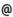## Babes-Bolyai University of Cluj-Napoca Faculty of Mathematics and Computer Science Study Cycle: Master SUBJECT

 Code Subject MMC1008 Efficient Numerical Models in Computational Sciences
 Section Semester Hours: C+S+L Category Type Modeling and Simulation - in English 2 2+1+1 speciality compulsory
 Teaching Staff in Charge
 Assoc.Prof. CHIOREAN Ioana Rodica, Ph.D.,  ioanacs.ubbcluj.ro
 Aims The purpose of this course is to aquainted the students with numerical methods for solving problems arising in Biology, Genetics, Mathematical Finance, etc. A study of efficiency of the presented methods, by comparing serial and parallel algorithms, will be made.
 Content 1. The DNA molecules, which are the most important carrier of genetic material, are sequences defined on an alphabet composed by the letters A,G,C and T which represent the four nucleotides that enter in their composition. So, the study of biological sequences consists in the study of sequences in general, informatics in particular. Under this circumstances, we take into account: -sequences comparison -the fitting or not fitting of some subsequences in long genetical sequences -the insertion of some subsequences in given sequences etc. The methods involved: dinamic programming, prefix computation, etc. Due to the fact that the biological sequences are very long, the problem of reducing the execution time is very important. In order to solve this, some parallel algorithms are presented. 2. Many problems in Finance are mathematicaly modeled by means of differential equations. For instance, the problem of determining the value of an option is modeled by means of the Black-Scholes equation. The study of some numerical methods in this domain implies: -numerical methods for discretizing differential equations -the Black-Scholes method -serial and parallel algorithms for solving exactly and approximatly the Black-Scholes equation -compariosn among the presented algorithms etc.
 References 1. Chiorean, I., Parallel Prefix Computation in Biological Sequences Comparison using Linear Recurrence Relations, Proceedings of the Mediteranean Conference on Medical and Biological Engineering, MEDICON 2004, Ischia (Italia), 31 iul-5 aug, 2004 2. Chiorean, I., Remarks on some Parallel Algorithms for Prefix Computation, International Journal of Pure and Applied Mathematics, vol.18, no.3, 2005, pp.363-369. 3. Chiorean, I., On some Numerical Methods for Solving the Black-Scholes Formula, Creative mathematics journal, vol.13, 2004, Pub.by Dep.of Math.and Comp.Science, North Univ.Baia-Mare, pp.31-36 (conf.ICAM4, Suior, Baia-Mare) 4. Chiorean, I., On the Complexity of some Parallel Algorithms for Biological sequences Comparison, Proc.of MEDINF 2003 Int.Conference, Craiova, 2003, pp.117-118 5. Chiorean, I., Calcul paralel, Ed.Microinformatica, 1994 6. Berger, B., Introduction to Computational Molecular Biology, MIT comp Biology Ed., 1998 7. Etheridge, A., A Course in Financial Calculus, University Oxford
 Assessment 50% seminar activity, 50% test paper
 Links: Syllabus for all subjects Romanian version for this subject Rtf format for this subject